# The GLM Procedure

### Parameterization of PROC GLM Models

Subsections:

The GLM procedure constructs a linear model according to the specifications in the MODEL statement. Each effect generates one or more columns in a design matrix. This section shows precisely howis built.

#### Intercept

All models include a column of 1s by default to estimate an intercept parameter. You can use the NOINT option to suppress the intercept.

#### Regression Effects

Regression effects (covariates) have the values of the variables copied into the design matrix directly. Polynomial terms are multiplied out and then installed in.

#### Main Effects

If a classification variable has m levels, PROC GLM generates m columns in the design matrix for its main effect. Each column is an indicator variable for one of the levels of the classification variable. The default order of the columns is the sort order of the values of their levels; this order can be controlled with the ORDER= option in the PROC GLM statement, as shown in the following table.

 Data Design Matrix A B A BA1 A2 B1 B2 B3 1 1 1 1 0 1 0 0 1 2 1 1 0 0 1 0 1 3 1 1 0 0 0 1 2 1 1 0 1 1 0 0 2 2 1 0 1 0 1 0 2 3 1 0 1 0 0 1

There are more columns for these effects than there are degrees of freedom for them; in other words, PROC GLM is using an over-parameterized model.

#### Crossed Effects

First, PROC GLM reorders the terms to correspond to the order of the variables in the CLASS statement; thus, B*A becomes A*B if A precedes B in the CLASS statement. Then, PROC GLM generates columns for all combinations of levels that occur in the data. The order of the columns is such that the rightmost variables in the cross index faster than the leftmost variables. No columns are generated corresponding to combinations of levels that do not occur in the data.

 Data Design Matrix A B A*B A BA1 A2 B1 B2 B3 A1B1 A1B2 A1B3 A2B1 A2B2 A2B3 1 1 1 1 0 1 0 0 1 0 0 0 0 0 1 2 1 1 0 0 1 0 0 1 0 0 0 0 1 3 1 1 0 0 0 1 0 0 1 0 0 0 2 1 1 0 1 1 0 0 0 0 0 1 0 0 2 2 1 0 1 0 1 0 0 0 0 0 1 0 2 3 1 0 1 0 0 1 0 0 0 0 0 1

In this matrix, main-effects columns are not linearly independent of crossed-effect columns; in fact, the column space for the crossed effects contains the space of the main effect.

#### Nested Effects

Nested effects are generated in the same manner as crossed effects. Hence, the design columns generated by the following statements are the same (but the ordering of the columns is different):

 model y=a b(a); (B nested within A) model y=a a*b; (omitted main effect for B)

The nesting operator in PROC GLM is more a notational convenience than an operation distinct from crossing. Nested effects are characterized by the property that the nested variables never appear as main effects. The order of the variables within nesting parentheses is made to correspond to the order of these variables in the CLASS statement. The order of the columns is such that variables outside the parentheses index faster than those inside the parentheses, and the rightmost nested variables index faster than the leftmost variables.

 Data Design Matrix A B(A) A BA1 A2 B1A1 B2A1 B3A1 B1A2 B2A2 B3A2 1 1 1 1 0 1 0 0 0 0 0 1 2 1 1 0 0 1 0 0 0 0 1 3 1 1 0 0 0 1 0 0 0 2 1 1 0 1 0 0 0 1 0 0 2 2 1 0 1 0 0 0 0 1 0 2 3 1 0 1 0 0 0 0 0 1

#### Continuous-Nesting-Class Effects

When a continuous variable nests with a classification variable, the design columns are constructed by multiplying the continuous values into the design columns for the class effect.

 Data Design Matrix A X(A) X AA1 A2 X(A1) X(A2) 21 1 1 1 0 21 0 24 1 1 1 0 24 0 22 1 1 1 0 22 0 28 2 1 0 1 0 28 19 2 1 0 1 0 19 23 2 1 0 1 0 23

This model estimates a separate slope for X within each level of A.

#### Continuous-by-Class Effects

Continuous-by-class effects generate the same design columns as continuous-nesting-class effects. The two models differ by the presence of the continuous variable as a regressor by itself, in addition to being a contributor to X*A.

 Data Design Matrix A X*A X AX A1 A2 X*A1 X*A2 21 1 1 21 1 0 21 0 24 1 1 24 1 0 24 0 22 1 1 22 1 0 22 0 28 2 1 28 0 1 0 28 19 2 1 19 0 1 0 19 23 2 1 23 0 1 0 23

Continuous-by-class effects are used to test the homogeneity of slopes. If the continuous-by-class effect is nonsignificant, the effect can be removed so that the response with respect to X is the same for all levels of the classification variables.

#### General Effects

An example that combines all the effects is

X1*X2*A*B*C(D E)

The continuous list comes first, followed by the crossed list, followed by the nested list in parentheses.

The sequencing of parameters is important to learn if you use the CONTRAST or ESTIMATE statement to compute or test some linear function of the parameter estimates.

Effects might be retitled by PROC GLM to correspond to ordering rules. For example, B*A(E D) might be retitled A*B(D E) to satisfy the following:

• Classification variables that occur outside parentheses (crossed effects) are sorted in the order in which they appear in the CLASS statement.

• Variables within parentheses (nested effects) are sorted in the order in which they appear in a CLASS statement.

The sequencing of the parameters generated by an effect can be described by which variables have their levels indexed faster:

• Variables in the crossed part index faster than variables in the nested list.

• Within a crossed or nested list, variables to the right index faster than variables to the left.

For example, suppose a model includes four effects—A, B, C, and D—each having two levels, 1 and 2. If the CLASS statement is

class A B C D;


then the order of the parameters for the effect B*A(C D), which is retitled A*B(C D), is as follows.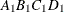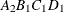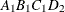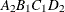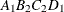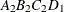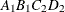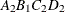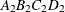Note that first the crossed effects B and A are sorted in the order in which they appear in the CLASS statement so that A precedes B in the parameter list. Then, for each combination of the nested effects in turn, combinations of A and B appear. The B effect changes fastest because it is rightmost in the (renamed) cross list. Then A changes next fastest. The D effect changes next fastest, and C is the slowest since it is leftmost in the nested list.

When numeric classification variables are used, their levels are sorted by their character format, which might not correspond to their numeric sort sequence. Therefore, it is advisable to include a format for numeric classification variables or to use the ORDER= INTERNAL option in the PROC GLM statement to ensure that levels are sorted by their internal values.

#### Degrees of Freedom

For models with classification (categorical) effects, there are more design columns constructed than there are degrees of freedom for the effect. Thus, there are linear dependencies among the columns. In this event, the parameters are not jointly estimable; there is an infinite number of least squares solutions. The GLM procedure uses a generalized-inverse to obtain values for the estimates; see the section Computational Method for more details. The solution values are not produced unless the SOLUTION option is specified in the MODEL statement. The solution has the characteristic that estimates are zero whenever the design column for that parameter is a linear combination of previous columns. (Strictly termed, the solution values should not be called estimates, since the parameters might not be formally estimable.) With this full parameterization, hypothesis tests are constructed to test linear functions of the parameters that are estimable.

Other procedures (such as the CATMOD procedure) reparameterize models to full rank by using certain restrictions on the parameters. PROC GLM does not reparameterize, making the hypotheses that are commonly tested more understandable. See Goodnight (1978a) for additional reasons for not reparameterizing.

PROC GLM does not actually construct the entire design matrix; rather, a rowofis constructed for each observation in the data set and used to accumulate the crossproduct matrix.